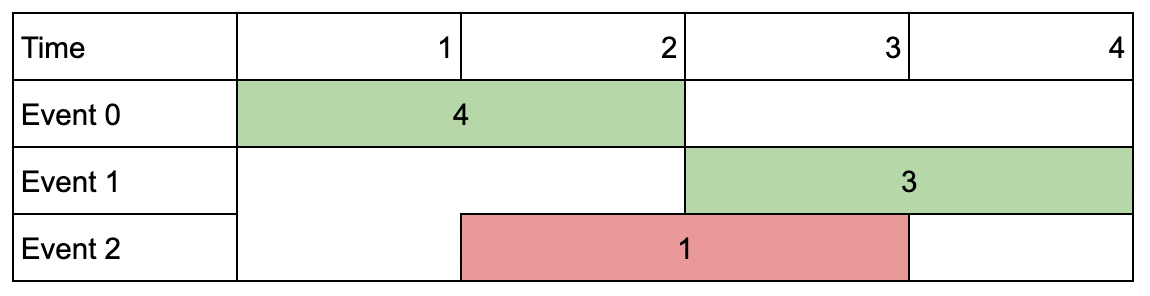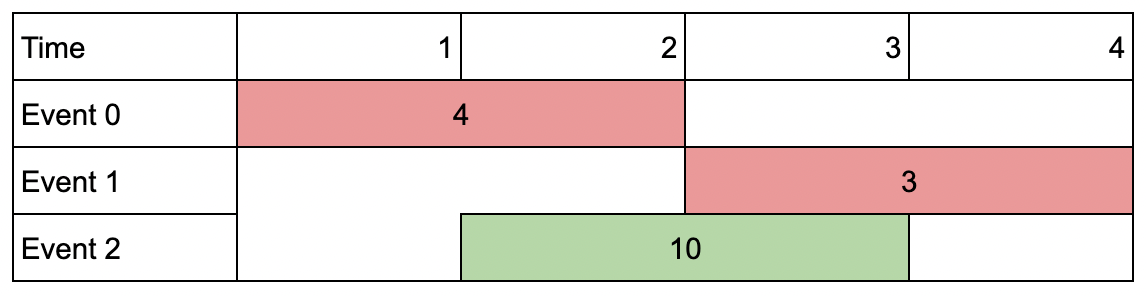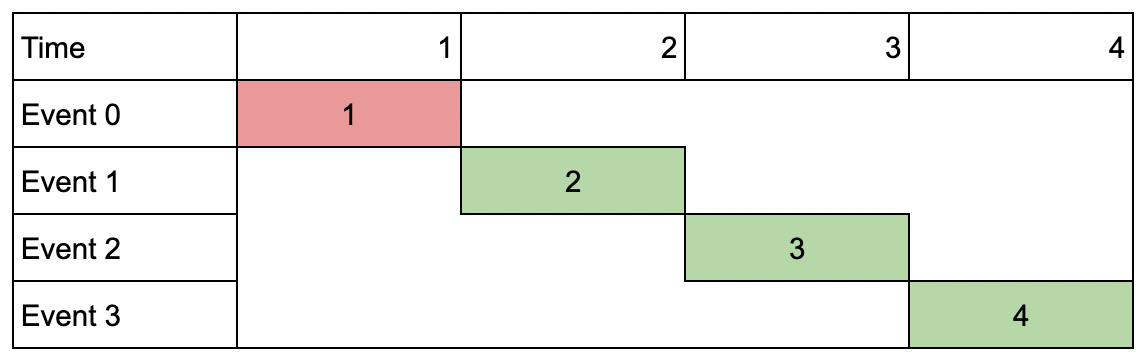Formatted question description: https://leetcode.ca/all/1751.html

# 1751. Maximum Number of Events That Can Be Attended II

Hard

## Description

You are given an array of events where events[i] = [startDay_i, endDay_i, value_i]. The i-th event starts at startDay_i and ends at endDay_i, and if you attend this event, you will receive a value of value_i. You are also given an integer k which represents the maximum number of events you can attend.

You can only attend one event at a time. If you choose to attend an event, you must attend the entire event. Note that the end day is inclusive: that is, you cannot attend two events where one of them starts and the other ends on the same day.

Return the maximum sum of values that you can receive by attending events.

Example 1:Input: events = [[1,2,4],[3,4,3],[2,3,1]], k = 2

Output: 7

Explanation: Choose the green events, 0 and 1 (0-indexed) for a total value of 4 + 3 = 7.

Example 2:Input: events = [[1,2,4],[3,4,3],[2,3,10]], k = 2

Output: 10

Explanation: Choose event 2 for a total value of 10.

Notice that you cannot attend any other event as they overlap, and that you do not have to attend k events.

Example 3:Input: events = [[1,1,1],[2,2,2],[3,3,3],[4,4,4]], k = 3

Output: 9

Explanation: Although the events do not overlap, you can only attend 3 events. Pick the highest valued three.

Constraints:

• 1 <= k <= events.length
• 1 <= k * events.length <= 10^6
• 1 <= startDay_i <= endDay_i <= 10^9
• 1 <= value_i <= 10^6

## Solution

First sort events according to the end days. Then use dynamic programming. Suppose there are n events. Create a 2D array dp of n rows and k + 1 columns, where dp[i][j] represents the maximum sum of values when considering the first i + 1 events where the maximum number of events that can be attended is j.

For the first event, dp = events. For the remaining events, first use binary search to find the maximum index of the previous event such that the previous event’s end day is before the current event, and then calculate the value in dp correspondingly.

Finally, return the maximum value in dp.

class Solution {
public int maxValue(int[][] events, int k) {
int maxValue = 0;
Arrays.sort(events, new Comparator<int[]>() {
public int compare(int[] event1, int[] event2) {
return event1 - event2;
}
});
int length = events.length;
int[][] dp = new int[length][k + 1];
int[] event0 = events;
for (int j = 1; j <= k; j++) {
dp[j] = event0;
maxValue = Math.max(maxValue, dp[j]);
}
for (int i = 1; i < length; i++) {
int[] event = events[i];
int startDay = event, value = event;
int prevIndex = binarySearch(events, startDay);
if (prevIndex < 0) {
for (int j = 1; j <= k; j++) {
dp[i][j] = Math.max(dp[i - 1][j], value);
maxValue = Math.max(maxValue, dp[i][j]);
}
} else {
for (int j = 1; j <= k; j++) {
dp[i][j] = Math.max(dp[i - 1][j], dp[prevIndex][j - 1] + value);
maxValue = Math.max(maxValue, dp[i][j]);
}
}
}
return maxValue;
}

public int binarySearch(int[][] events, int startDay) {
if (events >= startDay)
return -1;
int low = 0, high = events.length - 1;
while (low < high) {
int mid = (high - low + 1) / 2 + low;
int endDay = events[mid];
if (endDay >= startDay)
high = mid - 1;
else
low = mid;
}
return low;
}
}